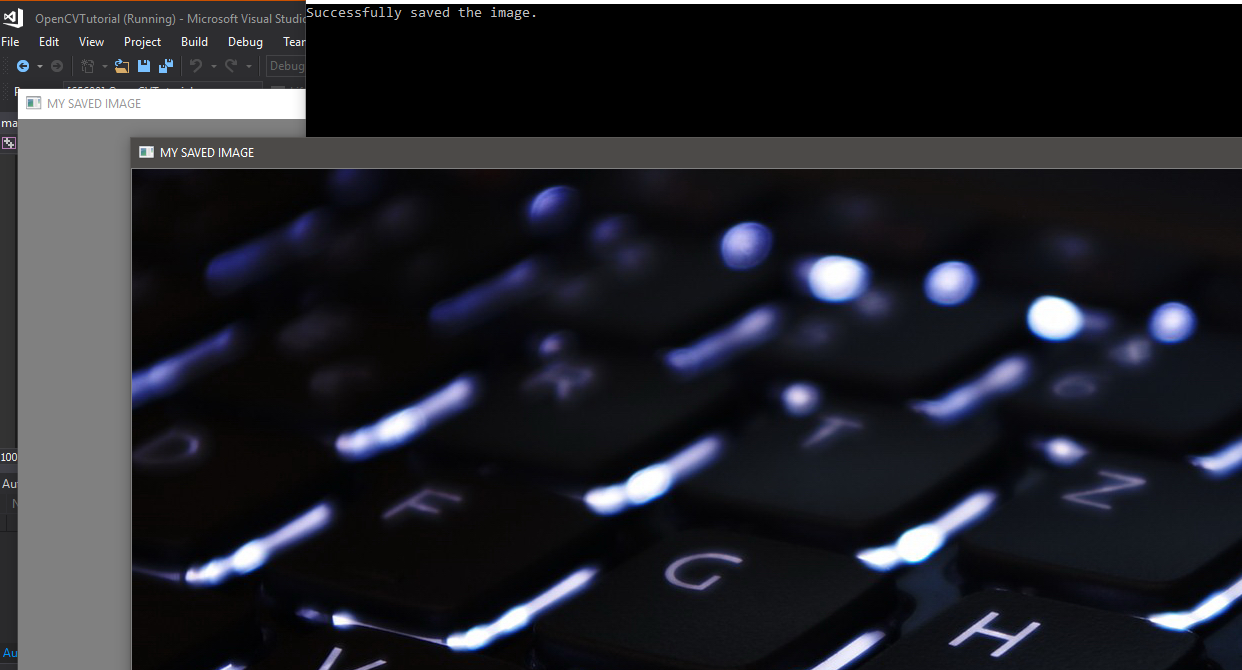GFG App
Open AppBrowser
Continue

# OpenCV | Saving an Image

This article aims to learn how to save an image from one location to any other desired location on your system in CPP using OpenCv. Using OpenCV, we can generate a blank image with any colour one wishes to.

So, let us dig deep into it and understand the concept with the complete explanation.

### Code : C++ code for saving an image to any location in OpenCV.

 `// c++ code explaining how to ` `// save an image to a defined ` `// location in OpenCV ` ` `  `// loading library files ` `#include ` `#include ` `#include ` ` `  `using` `namespace` `cv; ` `using` `namespace` `std; ` ` `  `int` `main(``int` `argc, ``char``** argv) ` `{ ` ` `  `    ``// Reading the image file from a given location in system ` `    ``Mat img = imread(``"..path\\abcd.jpg"``); ` ` `  `    ``// if there is no image ` `    ``// or in case of error ` `    ``if` `(img.empty()) { ` `        ``cout << ``"Can not open or image is not present"` `<< endl; ` ` `  `        ``// wait for any key to be pressed ` `        ``cin.get(); ` `        ``return` `-1; ` `    ``} ` ` `  `    ``// You can make any changes ` `    ``// like blurring, transformation ` ` `  `    ``// writing the image to a defined location as JPEG ` `    ``bool` `check = imwrite(``"..path\\MyImage.jpg"``, img); ` ` `  `    ``// if the image is not saved ` `    ``if` `(check == ``false``) { ` `        ``cout << ``"Mission - Saving the image, FAILED"` `<< endl; ` ` `  `        ``// wait for any key to be pressed ` `        ``cin.get(); ` `        ``return` `-1; ` `    ``} ` ` `  `    ``cout << ``"Successfully saved the image. "` `<< endl; ` ` `  `    ``// Naming the window ` `    ``String geek_window = ``"MY SAVED IMAGE"``; ` ` `  `    ``// Creating a window ` `    ``namedWindow(geek_window); ` ` `  `    ``// Showing the image in the defined window ` `    ``imshow(geek_window, img); ` ` `  `    ``// waiting for any key to be pressed ` `    ``waitKey(0); ` ` `  `    ``// destroying the creating window ` `    ``destroyWindow(geek_window); ` ` `  `    ``return` `0; ` `} `

Input :Output :### Explanation :

 `// Reading the image file from a given location in system ` `Mat img = imread(``"..path\\abcd.jpg"``); ` ` `  `// if there is no image ` `// or in case of error ` `if` `(img.empty()) { ` `    ``cout << ``"Can not open or image is not present"` `<< endl; ` ` `  `    ``// wait for any key to be pressed ` `    ``cin.get(); ` `    ``return` `-1; ` `} `

This part of the code reads the image from the path we have given to it. And it takes care of any error (if occurs). If there is no image present at this path, then “Can not open or image is not present” message will display and at the press of any key, the window will exit.

 `// writing the image to a defined location as JPEG ` `bool` `check = imwrite(``"..path\\MyImage.jpg"``, img); ` ` `  `// if the image is not saved ` `if` `(check == ``false``) { ` `    ``cout << ``"Mission - Saving the image, FAILED"` `<< endl; ` ` `  `    ``// wait for any key to be pressed ` `    ``cin.get(); ` `    ``return` `-1; ` `} ` ` `  `cout << ``"Successfully saved the image. "` `<< endl; `

This part of the code write the image to the defined path and if not successful, it will generate “Mission – Saving the image, FAILED” message and at the press of any key, the window will exit. And rest of the code will create the window and display the image in it. It will keep on displaying the image in the window until the key is pressed. Finally, the window will be destroyed.

My Personal Notes arrow_drop_up